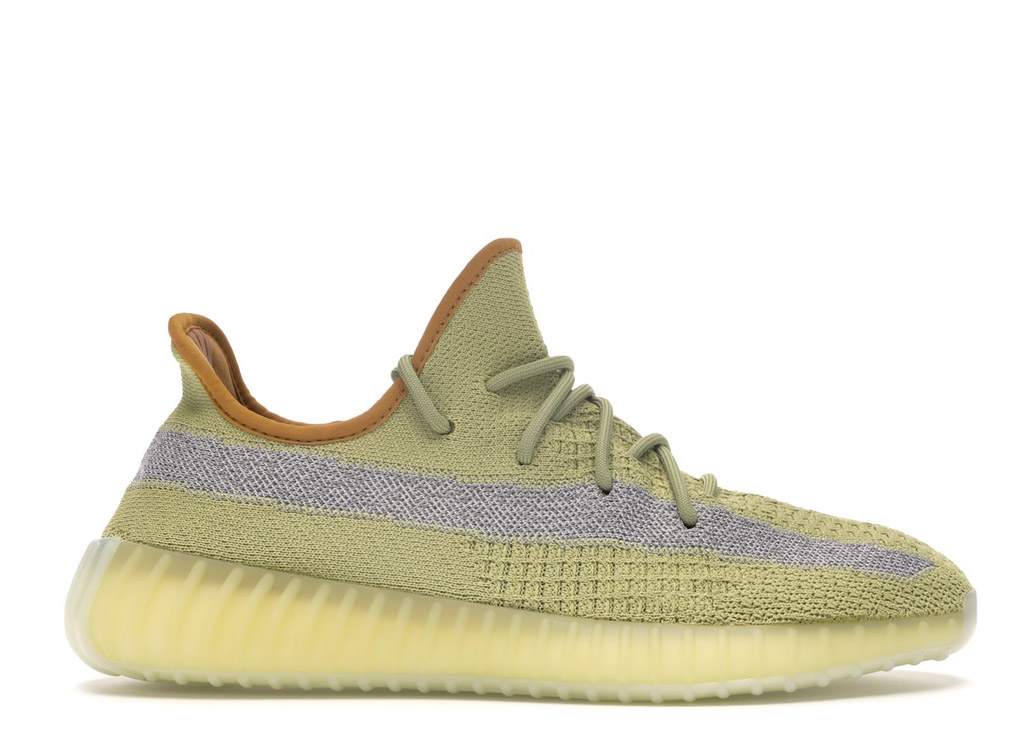All Categories
US\$

#### Cart

Home>Adidas>Yeezy Boost 350 V2>Yeezy Boost 350 V2 A1 Batch>2020 Yeezy 350 V2 Marsh Reflective Stripe(Ln5 A1)

# 2020 Yeezy 350 V2 Marsh Reflective Stripe(Ln5 A1)

US\$ 128.00
Quality Control:
QC by myself (1-3 days prepation time) QC by FKZ team (Get shipped faster)
US10.5=UK10=EU442/3=28.5
US\$ 128.00
US10=UK9.5=EU44=28CM
US\$ 128.00
US11.5=UK11=EU46=29.5CM
US\$ 128.00
US11=UK10.5=EU451/3=29CM
US\$ 128.00
US12.5=UK12=EU47.3=30.5CM
US\$ 128.00
US12=UK11.5=EU46.7=30CM
US\$ 128.00
US4.5=UK4=EU36.7=22.5CM
US\$ 128.00
US4=UK3.5=EU36=22CM
US\$ 128.00
US5.5=EUR38=UK5=23.5CM
US\$ 128.00
US5=UK4.5=EU37.5=23CM
US\$ 128.00
US6.5=UK6=EU39=24.5CM
US\$ 128.00
US6=UK5.5=EU38.5=24CM
US\$ 128.00
US7.5=UK7=EU402/3=25.5CM
US\$ 128.00
US7=UK6.5=EU40=25CM
US\$ 128.00
US8.5=UK8=EU42=26.5CM
US\$ 128.00
US8=UK7.5=EU411/3=26CM
US\$ 128.00
US9.5=UK9=EU431/3=27.5CM
US\$ 128.00
US9=UK8.5=EU422/3=27CM
US\$ 128.00
Quantity: 0 , Subtotal 0
• Detail

please contact with us to get our payment info, thank u flightkickz@gmail.com Made by Ln5 factory ,A1 final batch the quality is the best one in the market